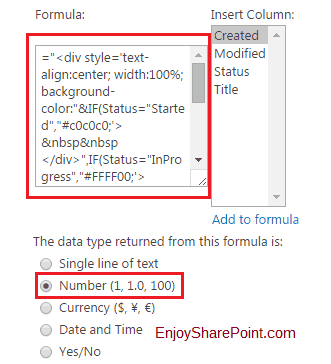# Color Code SharePoint List Column Value based on Condition using Calculated ColumnThis SharePoint tutorial, we will discuss how to color code SharePoint list column value based on condition using the calculated column in SharePoint.

## Show list column value in a different color in SharePoint

This example explains, how to show colors based on status column value in SharePoint 2013 list using calculated column.

Here I have a SharePoint list which has a choice column known as “Status” column and it has values like:

• Started
• InProgress
• Incomplete
• Critical
• Done

So based on this value we want to show different color in the list view so that by color it will be easy to differentiate from one another in the SharePoint list.

For this, we took a calculated column and in that calculated column we put the below logic like below:

``="<div style='text-align:center; width:100%; background-color:"&IF([Status]="Started","#c0c0c0;'> &nbsp&nbsp </div>",IF([Status]="InProgress","#FFFF00;'> &nbsp&nbsp </div>",IF([Status]="Incomplete","#FF9900 ;'>&nbsp&nbsp </div> ",IF([Status]="Critical","#FF0000;'> &nbsp&nbsp </div>",IF([Status]="Done","#00B050;'> &nbsp&nbsp </div>")))))``

And also make sure that you need to choose “Number” in the “The data type returned from this formula is:” section for the calculated column. It will look like below:

After this based on the status column in the list, the color will come like below:

You may like following SharePoint tutorials:

Hope this SharePoint tutorial explained, how to color-code SharePoint list column value based on condition using the calculated column in SharePoint.

Check out Best Alternative to InfoPath -> Try NowSharePoint Online FREE Training

JOIN a FREE SharePoint Video Course (3 Part Video Series)